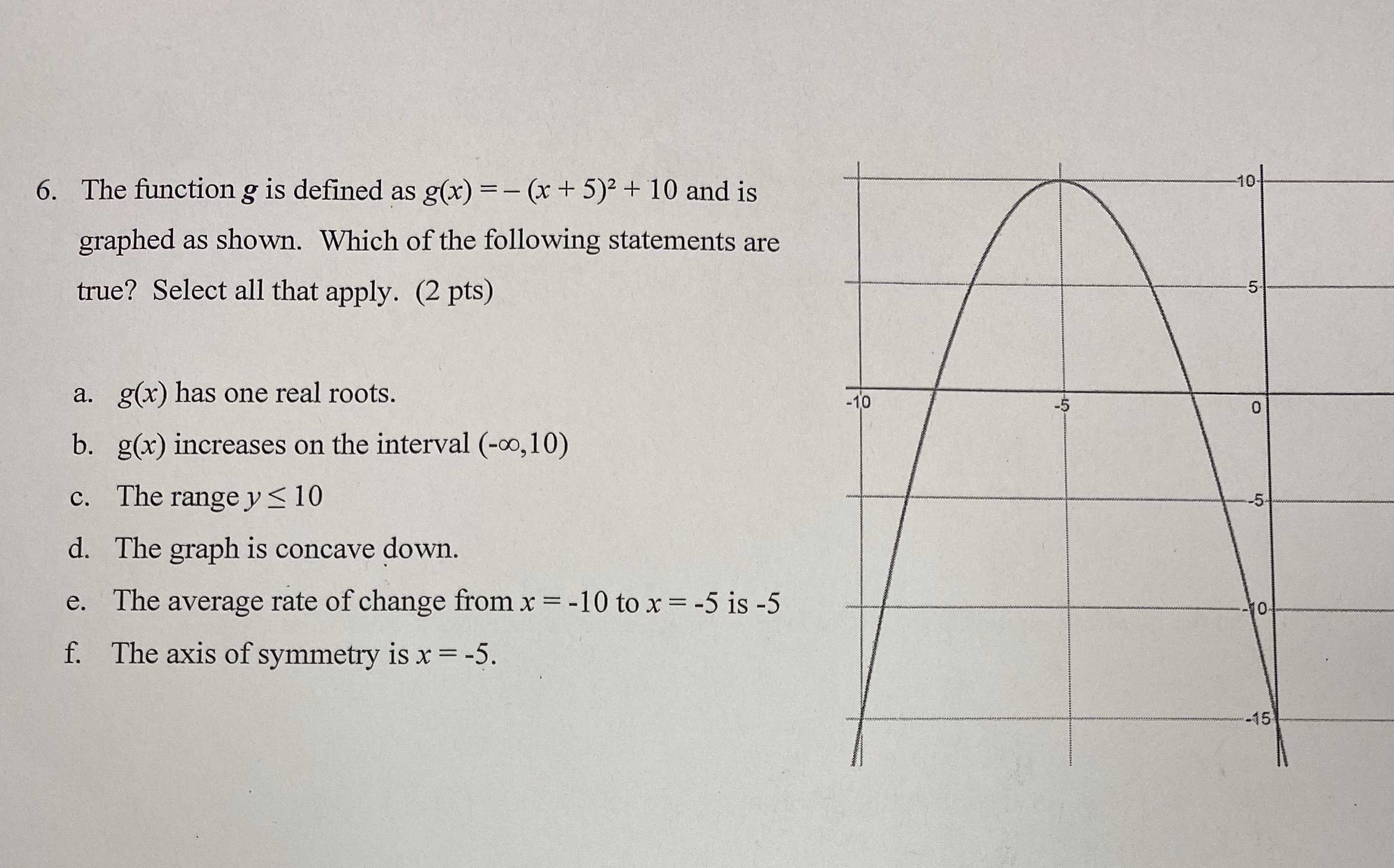### ¿Todavía tienes preguntas de matemáticas?

Pregunte a nuestros tutores expertos
Algebra
Pregunta6. The function $$g$$ is defined as $$g ( x ) = - ( x + 5 ) ^ { 2 } + 10$$ and is graphed as shown. Which of the following statements are true? Select all that apply. (2 pts)

a. $$g ( x )$$ has one real roots.

b. $$g ( x )$$ increases on the interval $$( - \infty , 10 )$$

c. The range $$y \leq 10$$

d. The graph is concave down. e. The average rate of change from $$x = - 10$$ to $$x = - 5$$ is $$- 5$$ f. The axis of symmetry is $$x = - 5$$ .